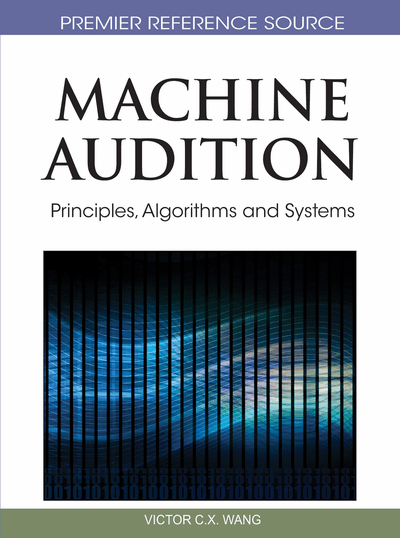# Tensor Factorization with Application to Convolutive Blind Source Separation of Speech

DOI: 10.4018/978-1-61520-919-4.ch008
Available
\$37.50
No Current Special Offers

## Abstract

Tensor factorization (TF) is introduced as a powerful tool for solving multi-way problems. As an effective and major application of this technique, separation of sound particularly speech signal sources from their corresponding convolutive mixtures is described and the results are demonstrated. The method is flexible and can easily incorporate all possible parameters or factors into the separation formulation. As a consequence of that fewer assumptions (such as uncorrelatedness and independency) will be required. The new formulation allows further degree of freedom to the original parallel factor analysis (PARAFAC) problem in which the scaling and permutation problems of the frequency domain blind source separation (BSS) can be resolved. Based on the results of experiments using real data in a simulated medium, it has been concluded that compared to conventional frequency domain BSS methods, both objective and subjective results are improved when the proposed algorithm is used.
Chapter Preview
Top

## Convolutive Blind Source Separation

The problem of convolutive BSS has been under research over the past two decades. A number of papers and reviews on convolutive BSS as addressed in (Pederson et al., 2007) have been published recently. In many practical situations the signals reach the sensors with different time delays. The corresponding delay between source j and sensor i, in terms of number of samples, is directly proportional to the sampling frequency and conversely to the speed of sound in the medium, i.e., where dij, fs, and c are respectively, the distance between source j and sensor i, the sampling frequency, and the speed of sound. For speech and music in the air as an example we may have dij in terms of meters, fs between 8 to 44 KHz, and c=330 m/sec. Also, in an acoustic environment the sound signals can reach the sensors through multi-paths after reflections by obstacles (such as walls). A general matrix formulation of the CBSS for mixing and separating the source signals can be given as:

(1) and(2) where M × 1 s(t), N × 1 x(t), and N × 1 v(t) denote respectively the vector of source signals, observed signals, and noise at discrete time t.H is the mixing matrix of size N × M and * denotes convolution operator. The separation is performed by means of a separating M × N matrix, W, which uses only the information about x(t) to reconstruct the original source signals denoted as y(t).

## Complete Chapter List

Search this Book:
Reset